Probability Symbols and Statistics Symbols

Probability Symbols and Statistics Symbols - Definition with Example

Probability deals with predicting the likelihood of an event. Many events cannot be predicted with total certainty, so the best we can say is how likely they are to happen, using the idea of probability. Probability is primarily a branch of mathematics, which studies the consequences of mathematical definitions and real-life entities.The probability of an event is expressed as a number 0 and 1, 0 indicates the impossibility and 1 indicates the certainty of an event. The higher the probability shows that the more likely it is that the event will occur. A simple example is the tossing of an unbiased coin. Since the coin is unbiased, there are two probable outcomes, either its heads or tails; the probability of “heads” is equal to the probability of “tails”; there is no other outcomes are possible, assuming the coin lands flat. So the probability of either “heads” or “tails” is ½ or 0.5 or 50%. An event having the probability of 0.5 is considered to have equal odds of occurring and no occurring. The probability that the coin will land without either side facing up is 0, because either "heads" or "tails" must be facing up.

Calculating probabilities in a situation like a coin toss is upfront, because the outcome of the coin lands without either of the sides facing up is 0. Each coin toss is an independent event; the outcome of one trial has no effect on following ones. No matter how many times one side lands facing up, the probability that it will do so at the next toss is always 0.5 (50%). The mistaken idea that a number of consecutive results (seven "heads" for example) makes it more likely that the next toss will result in a "tails" is known as the gambler's fallacy, one that has led to the downfall of many a bettor’s.

Probability theory had its start in the 17th century, when two French mathematicians, Blaise Pascal and Pierre de Fermat carried on a correspondence discussing mathematical problems dealing with the games of chance. The modern applications of probability theory run on the extent of human inquiry, and include aspects of computer programming, astrophysics, music, weather prediction, risk management, market assessment, entitlement analysis, environmental regulation, and financial regulation and medicine.

In order to measure probabilities, mathematicians devised the following formula to find probability of an event:

Probability of an Event Happening

$P(A)\:=\frac{Total\:Number\:of\:Ways\:Event\:"A"\:Can\:Occur}{Total\:Number\:of\:Possible\:Outcomes}$

Or in other way in its simplest form, probability can be expressed as the total number of occurrences of a targeted event divided by the total number of occurrences plus the total number of failures (this adds up to the total of possible outcomes):

P(A) = P(a)/[P(a) + P(b)]

Statistics

Statistics is a form of mathematical analysis for a given set of data or real-life studies that uses quantified models, representations and synopses to reach the results. Statistics studies methodologies to gather, review, analyze and draw conclusions from the experimental dataset. Some statistical measures include mean, mode, median, regression analysis, skewness, kurtosis, variance, and analysis of variance.

Understanding Statistics

Statistics is a term used to summarize a process that is used to characterize a data set. If the data set depends on a sample of a larger population, then one can develop interpretations about the population primarily based on the statistical outcome from the samples. Statistical analysis involves the process of gathering, reviewing, evaluating data and then summarizing the data into a mathematical form or statistical outcome.

More generally statistical methods are used to analyze large volumes of data and their properties.

Statistics is used in various disciplines such as psychology, business, social sciences, humanities, government, medical and manufacturing. Statistical data is gathered using a sample procedure. There are two types of statistical methods that are used in analyzing data: descriptive statistics and inferential statistics. Descriptive statistics are used to summarize data from a sample exercising the mean or standard deviation. Inferential statistics are used when data is considered as a sub-class of a specific population.

Types of Statistics

Statistics is a general, broad term, so it is natural that inside that umbrella there exist a number of different models.
Mean: A mean is the mathematical average of a group of two or more numbers. The mean for a specified set of numbers can be computed in multiple ways, including the arithmetic mean, which shows how well a specific commodity performs over time, and the geometric mean, which shows the performance results of an investor’s share invested in that same commodity over the same period.

Regression Analysis: Regression analysis determines the point to which specific factors such as interest rates, the price of a products or services, or particular industries influence the price variations of an asset. This is portrayed in the form of a straight line called linear regression line.

Skewness: The degree of a set of experimental data in which the data varies from the standard distribution is known as skewness. In case of most of the data sets, like stock prices and commodity returns, the data sets have either positive skew, a curve slanted toward the left of the data average, or negative skew, a curve slanted toward the right of the data average.
Kurtosis: Kurtosis measures whether the experimental data is light-tailed (less outlier-prone) or heavy-tailed (more outlier-prone) than the normal distribution. Data sets with high kurtosis have heavy tails, or outliers, which implies greater investment risk in the form of occasional wild returns. Data sets with low kurtosis have light tails, or lack of outliers, which implies lesser investment risk.

Variance: The measurement of the span between the numbers in a data set is called Variance. The variance measures the distance of each and every number in the data set through its mean. Variance can help to determine the risk an investor might accept when buying an investment plan.

Statistical Symbols

Probability and statistics symbols table and definitions.

Probability and statistics symbols table

 Symbol Symbol Name Meaning / definition Example P(A) Probability Function Probability of an event A P(A) = 0.25 P(A ∩ B) Probability of events Intersection Probability that of events A and B P(A∩B) = 0.35 P(A∪B) Probability of Events Union Probability that of events A or B P(A∪B) = 0.45 P(A | B) Conditional Probability Function Probability of event A, given event B occurred P(A | B) = 0.33 f (x) Probability Density Function (pdf) P(A≤ x ≤B) = ∫ f (x) dx F(x) Cumulative Distribution Function (cdf) F(x) = P(x >=X) Μ Population Mean Average of a group of two or more numbers. μ = 40 var(X) Variance Variance of random variable X var(X) = 4 σ2 Variance Variance of population values σ2 = 16 std(X) Standard Deviation Standard deviation of random variable X std(X) = 4 σX Standard Deviation Standard deviation value of random variable X σX = 4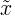Median Middle value of random variable x cov(X,Y) Covariance Covariance of random variables X and Y cov(X,Y) = 5 corr(X,Y) Correlation Correlation of random variables X and Y corr(X,Y) = 0.4 ρX,Y Correlation Correlation of random variables X and Y ρX,Y = 0.4 ∑ Summation Sum of all values in the range of series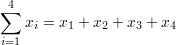∑∑ Double Summation Double summation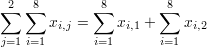Mo Mode Value that occurs most frequently in population Md Sample Median 1/2 the population is below this value Q1 Lower / First Quartile 1/4 of population are below this value Q2 Median / Second Quartile 1/2 of population are below this value = median of samples Q3 Upper / Third Quartile 3/4 of population are below this value

Combinatorics Symbols

 Symbol Symbol Name Meaning / definition Example n! Factorial n! = 1⋅2⋅3⋅...⋅n 4! = 1⋅2⋅3⋅4 = 24 nPk Permutation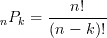4P3 = 4! / (4-3)! = 24 nCk Combination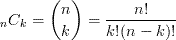4C3 = 4! / [3! (4-3)!]=4